$$\newcommand{L}{\| #1 \|}\newcommand{VL}{\L{ \vec{#1} }}\newcommand{R}{\operatorname{Re}\,(#1)}\newcommand{I}{\operatorname{Im}\, (#1)}$$

# The Fourier basis¶

Fourier without the ei shows that we can write the discrete Fourier transform DFT as vector dot products. In Rewriting the DFT with vectors we found that:

$X_k = \vec{x} \cdot \vec{c^k} - i \vec{x} \cdot \vec{s^k}$

where:

$\begin{split}\vec{r^k} = [ k 2 \pi \frac{0}{N}, k 2 \pi \frac{1}{N}, k 2 \pi \frac{N-1}{N}] \\ \vec{c^k} \triangleq \left[ \cos(r^k_0), \cos(r^k_1), \ldots \cos(r^k_{N-1} \right] \\ \vec{s^k} \triangleq \left[ \sin(r^k_0), \sin(r^k_1), \ldots \sin(r^k_{N-1} \right]\end{split}$

$$\vec{c_k}, \vec{s_k}$$ are Fourier basis vectors.

We can compile all these vectors into matrices to form Fourier basis matrices.

## Rewriting the DFT with cosine and sine basis matrices¶

Instead of writing the formulae for the individual elements $$X_k$$ and $$x_n$$, we can use matrices to express our formulae in terms of the vectors $$\vec{X}, \vec{x}$$.

$$\newcommand{C}{\mathbf{C}} \newcommand{S}{\mathbf{S}}$$ Define a matrix $$\C$$ that has rows $$[\vec{c_0}, \vec{c_1}, ..., \vec{c_{N-1}}]$$:

$\begin{split}\C \triangleq \begin{bmatrix} c_{0,0}, c_{0, 1}, ..., c_{0, N-1} \\ c_{1,0}, c_{1, 1}, ..., c_{1, N-1} \\ ... \\ c_{N-1,0}, c_{N-1, 1}, ..., c_{N-1, N-1} \\ \end{bmatrix}\end{split}$

Call $$\C$$ the cosine basis matrix.

Define a matrix $$\S$$ that has rows $$[\vec{s_0}, \vec{s_1}, ..., \vec{s_{N-1}}]$$:

$\begin{split}\S \triangleq \begin{bmatrix} s_{0,0}, s_{0, 1}, ..., s_{0, N-1} \\ s_{1,0}, s_{1, 1}, ..., s_{1, N-1} \\ ... \\ s_{N-1,0}, s_{N-1, 1}, ..., s_{N-1, N-1} \\ \end{bmatrix}\end{split}$

Call $$\S$$ the sine basis matrix.

Now we can rewrite the forward and inverse DFT as matrix products:

$\begin{split}\vec{X} = \C \cdot \vec{x} - i \S \cdot \vec{x} \\ \vec{x} = \frac{1}{N} \C \cdot \vec{X} + i \frac{1}{N} \S \cdot \vec{X}\end{split}$

>>> import numpy as np
>>> np.set_printoptions(precision=4, suppress=True)
>>> x = np.array(
...     [ 0.4967, -0.1383,  0.6477,  1.523 , -0.2342, -0.2341,  1.5792,
...       0.7674, -0.4695,  0.5426, -0.4634, -0.4657,  0.242 , -1.9133,
...      -1.7249, -0.5623, -1.0128,  0.3142, -0.908 , -1.4123,  1.4656,
...      -0.2258,  0.0675, -1.4247, -0.5444,  0.1109, -1.151 ,  0.3757,
...      -0.6006, -0.2917, -0.6017,  1.8523])
>>> N = len(x)


In order to run the commands in this page, you will need to download the file dft_plots.py to the directory where you running the code.

>>> import matplotlib.pyplot as plt
>>> import dft_plots as dftp


Hint

If running in the IPython console, consider running %matplotlib to enable interactive plots. If running in the Jupyter Notebook, use %matplotlib inline.

## Some properties of the cosine and sine basis matrices¶

First we note that $$\C$$ and $$\S$$ are always real matrices, regardless of the input $$\vec{x}$$ or $$\vec{X}$$.

Let’s show $$\C$$ and $$\S$$ as grayscale images.

First we build $$\C$$ and $$\S$$ for our case with $$N=32$$:

>>> C = np.zeros((N, N))
>>> S = np.zeros((N, N))
>>> ns = np.arange(N)
>>> one_cycle = 2 * np.pi * ns / N
>>> for k in range(N):
...     t_k = k * one_cycle
...     C[k, :] = np.cos(t_k)
...     S[k, :] = np.sin(t_k)

>>> fig, axes = plt.subplots(1, 2, figsize=(10, 5))
>>> dftp.show_array(axes, dftp.scale_array(C))
>>> axes.set_title("$\mathbf{C}$")
<...>
>>> dftp.show_array(axes, dftp.scale_array(S))
>>> axes.set_title("$\mathbf{S}$")
<...>


(png, hires.png, pdf)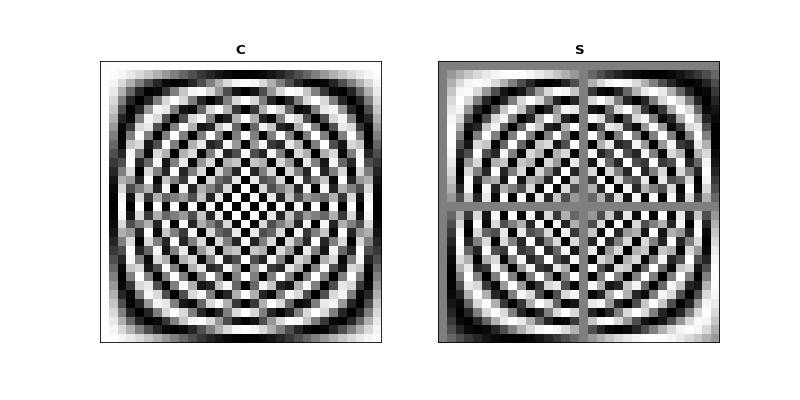### Mirror symmetry¶

From the images we see that the bottom half of $$\C$$ looks like a mirror image of the top half of $$\C$$. The bottom half of $$\S$$ looks like a sign flipped (black $$\Leftrightarrow$$ white) mirror image of the top half of $$\S$$. In fact this is correct:

$\begin{split}C_{p,:} = C_{N-p,:} \; \mathrm{for} \; p > 0 \\ S_{p,:} = -S_{N-p,:} \; \mathrm{for} \; p > 0\end{split}$

Why is this? Let’s look at lines from the center of $$\C$$. Here we are plotting the continuous cosine function with dotted lines, with filled circles to represent the discrete samples we took to fill the row of $$\C$$:

>>> center_rows = [N / 2. - 1, N / 2., N / 2. + 1]
>>> fig = dftp.plot_cs_rows('C', N, center_rows)
>>> fig.suptitle('Rows $N / 2 - 1$ through $N / 2 + 1$ of $\mathbf{C}$',
...              fontsize=20)
<...>


(png, hires.png, pdf)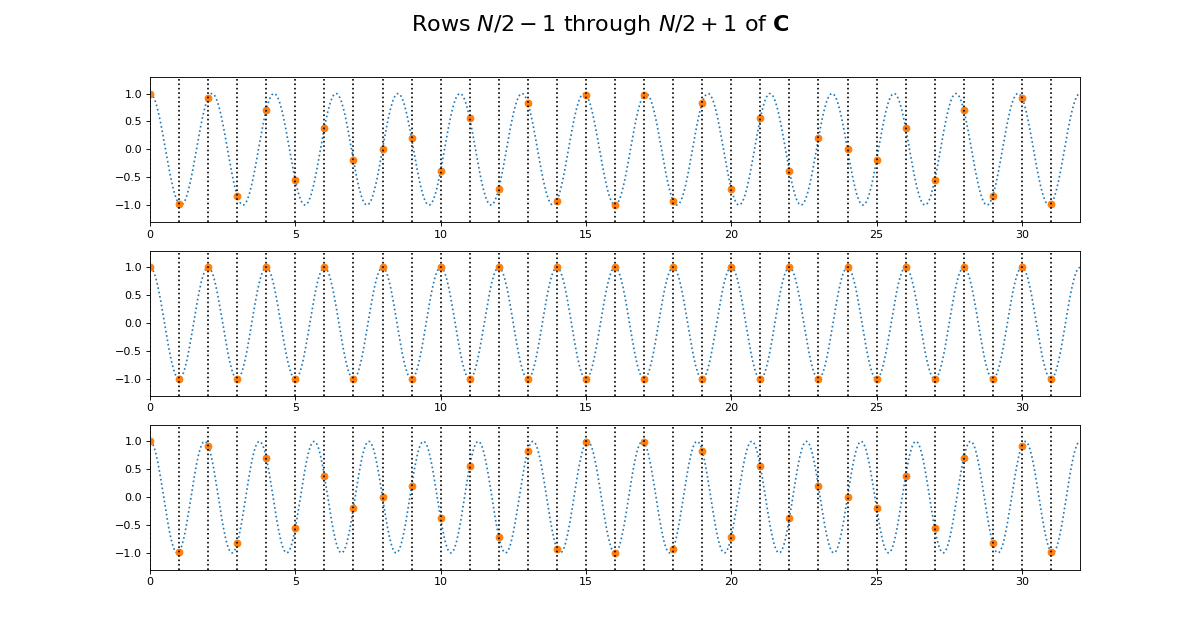The first plot in this grid is for row $$k = N / 2 - 1$$. This row starts sampling just before the peak and trough of the cosine. In the center is row $$k = N / 2$$ of $$\C$$. This is sampling the cosine wave exactly at the peak and trough. When we get to next row, at $$k = N / 2 + 1$$, we start sampling after the peak and trough of the cosine, and these samples are identical to the samples just before the peak and trough, at row $$k = N / 2 - 1$$. Row $$k = N / 2$$ is sampling at the Nyquist sampling frequency, and row $$k = N / 2 + 1$$ is sampling at a frequency lower than Nyquist and therefore it is being aliased to the same apparent frequency as row $$k = N / 2 - 1$$.

This might be more obvious plotting rows 1 and N-1 of $$\C$$:

>>> fig = dftp.plot_cs_rows('C', N, [1, N-1])
>>> fig.suptitle('Rows $1$ and $N - 1$ of $\mathbf{C}$',
...              fontsize=20)
<...>


(png, hires.png, pdf)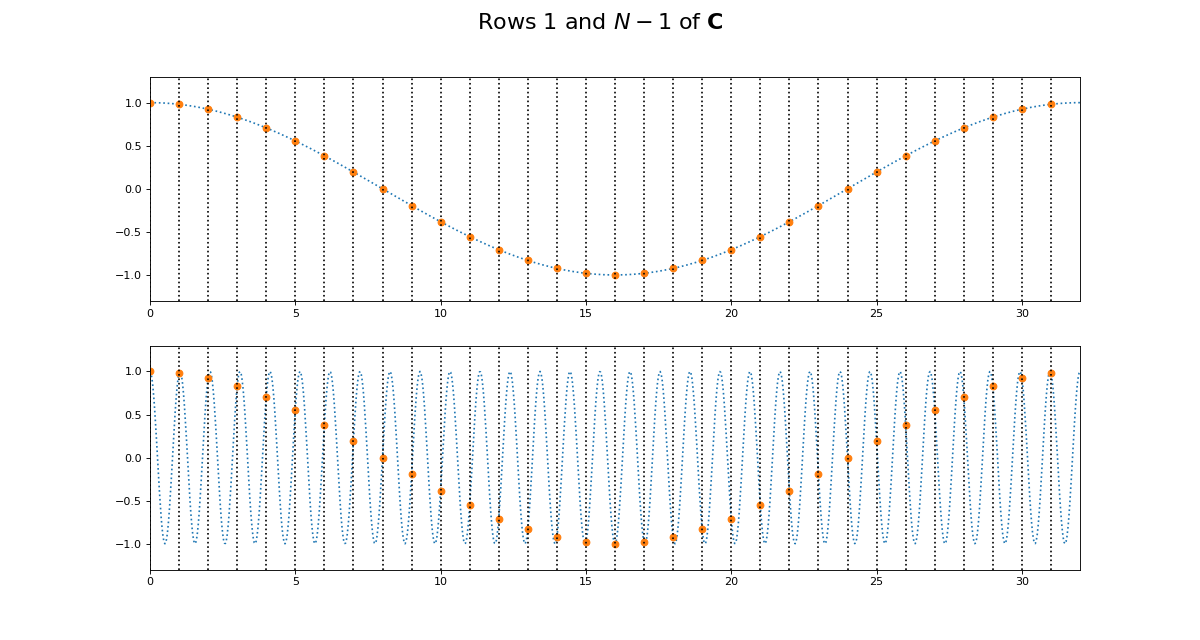Of course we get the same kind of effect for $$\S$$:

>>> fig = dftp.plot_cs_rows('S', N, center_rows)
>>> fig.suptitle('Rows $N / 2 - 1$ through $N / 2 + 1$ of $\mathbf{S}$',
...              fontsize=20)
<...>


(png, hires.png, pdf)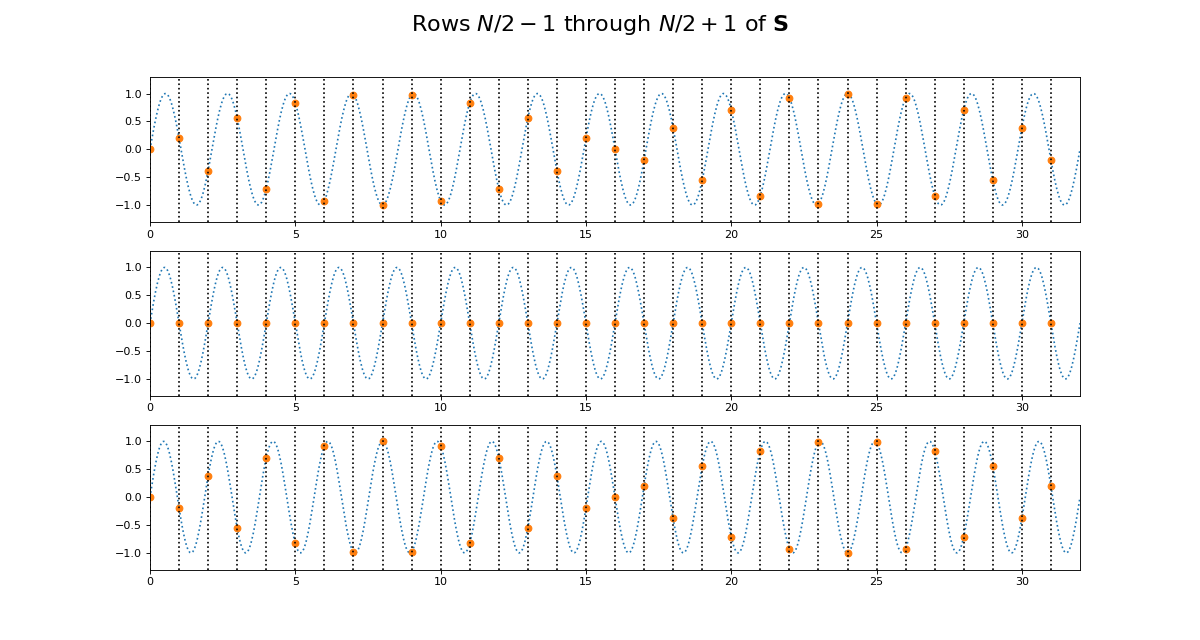>>> fig = dftp.plot_cs_rows('S', N, [1, N-1])
>>> fig.suptitle('Rows $1$ and $N - 1$ of $\mathbf{S}$',
...              fontsize=20)
<...>


(png, hires.png, pdf)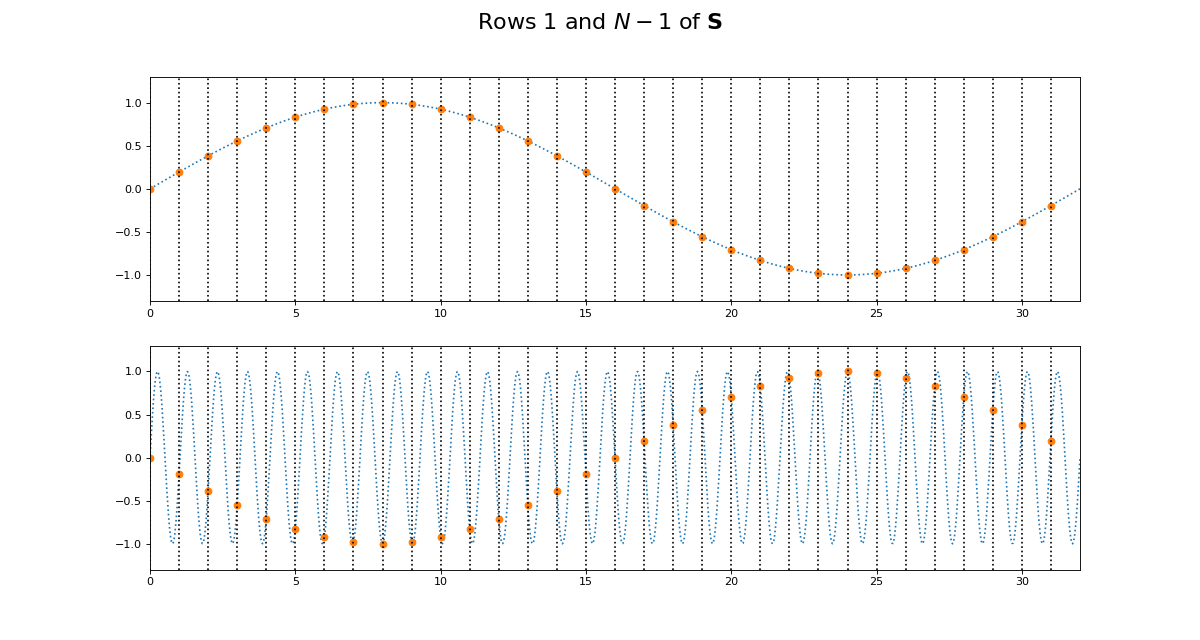Notice that for $$\S$$, the sine waves after $$k = N / 2$$ are sign-flipped relative to their matching rows before $$k = N / 2$$. Thus row $$k = N / 2 + 1$$ will be aliased to the same frequency as for row $$k = N / 2 - 1$$, but with a negative sign.

It is this sign-flip that leads to the concept of negative frequency in the DFT, and to the property of conjugate symmetry from the DFT on a vector of real numbers. We will hear more about these later.

### Matrix symmetry¶

The next thing we notice about $$\C$$ and $$\S$$ is that they are transpose symmetric matrices:

$\begin{split}\C = \C^T \\ \S = \S^T \\\end{split}$
>>> assert np.allclose(C, C.T)
>>> assert np.allclose(S, S.T)


Why is this? Consider the first column of $$\C$$. This is given by $$\cos(k 2 \pi 0 / N) = \cos(0)$$, and thus, like the first row of $$\C$$, is always = 1.

Now consider the second row of $$\C$$. This is a cosine sampled at horizontal axis values:

$\vec{t_1} \triangleq \left[ 2 \pi \frac{n}{N} \;\mathrm{for}\; n \in 0,1,\ldots,N-1 \right]$

Call $$t_{k, n}$$ the value of $$\vec{t_k}$$ at index $$n$$. Now consider the second column of $$\C$$. This is a cosine sampled at horizontal axis values for $$n = 1$$:

$\begin{split}t_{0,1} = (0) 2 \pi \frac{1}{N} \\ t_{1,1} = (1) 2 \pi \frac{1}{N} \\ ... \\ t_{N-1,1} = (N-1) 2 \pi \frac{1}{N} \\\end{split}$

In general, because the sequence $$k 0,1,,N-1$$ is equal to the sequence $$n \in 0,1,\ldots,N-1$$, this means that the column sampling positions for row $$n \in t_{0, n}, t_{1, n}, ... , t_{N-1, n}$$ are equal to the row sampling positions for corresponding ($$k = n$$) row $$k \in t_{k, 0}, t_{k, 1}, ... , t_{k, N-1}$$. Write column $$z$$ of $$\C$$ as $$C_{:,z}$$; column $$z$$ of $$\S$$ is $$S_{:, z}$$. Therefore $$C_{z, :} = C_{:, z}, S_{z, :} = S_{:, z}$$.

### Row dot products and lengths¶

It is useful to look at the dot products of the rows of $$\C$$ and $$\S$$. The dot product of each row with itself gives the squared length of the vector in that row.

The vector length of a vector $$\vec{v}$$ with $$N$$ elements is written as $$\| \vec{v} \|$$, and defined as:

$\| \vec{v} \| \triangleq \sqrt{\sum_{n=0}^{N-1} v_n^2} = \sqrt{ \vec{v} \cdot \vec{v} }$

The dot products of different rows of $$\C$$ and $$\S$$ give an index of the strength of the relationship between the rows. We can look at the dot products of all the rows of $$\C$$ with all other rows with the matrix multiplication $$\C^T \C$$:

>>> dftp.show_array(plt.gca(), dftp.scale_array(C.T.dot(C)))
>>> plt.title("$\mathbf{C^TC}$")
<...>


(png, hires.png, pdf)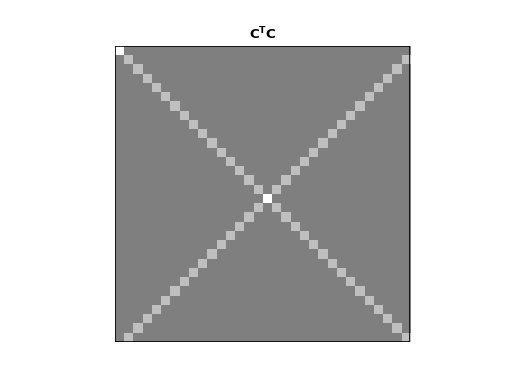The image shows us that the dot product between the rows of $$\C$$ is 0 everywhere except:

• the dot products of the rows with themselves (the squared vector lengths);
• the dot products of the mirror image vectors such as $$\vec{c_1}$$ and $$\vec{c_{N-1}}$$. Because $$\vec{c_n} = \vec{c_{N-n}}$$, these dot products are the same as the $$\| \vec{c_n} \|^2$$.

The squared row lengths are:

>>> np.diag(C.T.dot(C))
array([ 32.,  16.,  16.,  16.,  16.,  16.,  16.,  16.,  16.,  16.,  16.,
16.,  16.,  16.,  16.,  16.,  32.,  16.,  16.,  16.,  16.,  16.,
16.,  16.,  16.,  16.,  16.,  16.,  16.,  16.,  16.,  16.])


Notice that the rows $$\vec{c_0}$$ and $$\vec{c_{N / 2}}$$ have squared length $$N$$, and the other rows have squared length $$N / 2$$.

We can do the same for $$\S$$:

>>> dftp.show_array(plt.gca(), dftp.scale_array(S.T.dot(S)))
>>> plt.title("$\mathbf{S^TS}$")
<...>


(png, hires.png, pdf)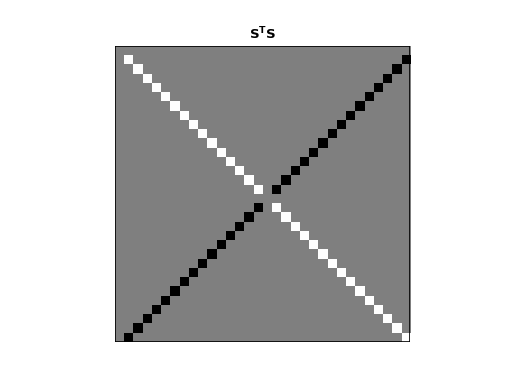Remember that $$\vec{s_0}$$ and $$\vec{s_{n/2}}$$ are all 0 vectors. The dot product of these rows with any other row, including themselves, is 0. All other entries in this $$\S^T \S$$ matrix are zero except:

• the dot products of rows with themselves (other than $$\vec{s_0}$$ and $$\vec{s_{n/2}}$$);
• the dot products of the flipped mirror image vectors such as $$\vec{s_1}$$ and $$\vec{s_{N-1}}$$. Because $$\vec{s_n} = -\vec{s_{N-n}}$$, these dot products are the same as $$-\| \vec{s_n} \|^2$$.

The squared row lengths are:

>>> np.diag(S.T.dot(S))
array([  0.,  16.,  16.,  16.,  16.,  16.,  16.,  16.,  16.,  16.,  16.,
16.,  16.,  16.,  16.,  16.,   0.,  16.,  16.,  16.,  16.,  16.,
16.,  16.,  16.,  16.,  16.,  16.,  16.,  16.,  16.,  16.])


The rows $$\vec{s_0}$$ and $$\vec{s_{N / 2}}$$ have squared length $$0$$, and the other rows have squared length $$N / 2$$.

Finally, let’s look at the relationship between the rows of $$\C$$ and the rows of $$\S$$:

>>> np.allclose(C.T.dot(S), 0)
True


The rows of $$\C$$ and $$\S$$ are completely orthogonal.

In fact these relationships hold for $$\C$$ and $$\S$$ for any $$N$$.

#### Proof for $$\C, \S$$ dot products¶

We can show these relationships with some more or less basic trigonometry.

Let’s start by looking at the dot product of two rows from $$\C$$. We will take rows $$\vec{c_p} =\C_{p,:}$$ and $$\vec{c_q} = \C_{q,:}$$. As we remember, these vectors are:

$\begin{split}\vec{c_p} = \left[ \cos(p n \frac{2 \pi}{N}) \;\mathrm{for}\; n \in 0,1,\ldots,N-1 \right] \\ \vec{c_q} = \left[ \cos(q n \frac{2 \pi}{N}) \;\mathrm{for}\; n \in 0,1,\ldots,N-1 \right]\end{split}$

So:

$\vec{c_p} \cdot \vec{c_q} = \sum_{n=0}^{N-1} \cos(p n \frac{2 \pi}{N}) \cos(q n \frac{2 \pi}{N})$

Our trigonometry tells us that:

$\cos \alpha \cos \beta = \frac{1}{2} [ \cos(\alpha + \beta) - \cos(\alpha - \beta) ]$

We can rewrite the dot product as the addition of two sums of cosines:

$\vec{c_p} \cdot \vec{c_q} = \frac{1}{2} \sum_{n=0}^{N-1} \cos((p + q) n \frac{2 \pi}{N}) + \frac{1}{2} \sum_{n=0}^{N-1} \cos((p - q) n \frac{2 \pi}{N})$

Now we can use the formulae for sums of arithmetic progressions of cosines and sines to solve these equations. Here are the formulae:

$\begin{split}R \triangleq \frac{\sin(N \frac{1}{2}d)}{\sin(\frac{1}{2} d)} \\ \sum_{n=0}^{N-1} \cos(a + nd) = \begin{cases} N \cos a & \text{if } \sin(\frac{1}{2}d) = 0 \\ R \cos ( a + (N - 1) \frac{1}{2} d) & \text{otherwise} \end{cases} \\ \sum_{n=0}^{N-1} \sin(a + nd) = \begin{cases} N \sin a & \text{if } \sin(\frac{1}{2}d) = 0 \\ R \sin ( a + (N - 1) \frac{1}{2} d) & \text{otherwise} \end{cases}\end{split}$

For our $$\C, \S$$ row dot product sums, starting angle $$a$$ is always 0, and the $$d$$ value in the formulae are always integer multiples of $$\frac{2 \pi}{N}$$. For example, $$d = (p \pm q) \frac{2 \pi}{N}$$ in the equations above. For our case, we can write $$d = g \frac{2 \pi}{N}$$ where $$g$$ is an integer.

$\begin{split}R = \frac{ \sin( g N \frac{1}{2} \frac{2 \pi}{N} ) } { \sin( g \frac{1}{2} \frac{2 \pi}{N} ) } \\ = \frac{ \sin( g \pi ) } { \sin( \frac{g}{N} \pi ) }\end{split}$

Because $$g$$ is an integer, the numerator of $$R$$ will always be 0, so the resulting sum is zero unless the denominator of $$R$$ is zero. The denominator is zero only if $$g$$ is a multiple of N, including 0. When the denominator is zero, the sum will be equal to $$N \cos(a) = N \cos(0) = N$$ for a cosine series or $$N \sin(a) = N \sin(0) = 0$$ for a sine series.

Now we can calculate our dot product:

$\begin{split}\vec{c_p} \cdot \vec{c_q} = \begin{cases} \frac{1}{2} N + \frac{1}{2} N = N & \text{if } p = q, p \in 0, N/2 \\ \frac{1}{2} N & \text{if } p = q, p \notin 0, N/2 \\ \frac{1}{2} N & \text{if } p + q = N, p \ne N/2 \\ 0 & \text{otherwise} \end{cases}\end{split}$

We can apply the same kind of logic to the rows of $$\S$$:

$\sin \alpha \sin \beta = \frac{1}{2} [ \cos(\alpha - \beta) - \cos(\alpha + \beta) ]$

So:

$\vec{s_p} \cdot \vec{s_q} = \frac{1}{2} \sum_{n=0}^{N-1} \cos((p - q) n \frac{2 \pi}{N}) - \frac{1}{2} \sum_{n=0}^{N-1} \cos((p + q) n \frac{2 \pi}{N})$

This gives:

$\begin{split}\vec{s_p} \cdot \vec{s_q} = \begin{cases} 0 & \text{if } p = q, p \in 0, N/2 \\ \frac{1}{2} N & \text{if } p = q, p \notin 0, N/2 \\ -\frac{1}{2} N & \text{if } p + q = N, p \ne N/2 \\ 0 & \text{otherwise} \end{cases}\end{split}$